# Test: Three Dimensional 3D Geometry (Competition Level) - 1

## 30 Questions MCQ Test Mathematics (Maths) Class 12 | Test: Three Dimensional 3D Geometry (Competition Level) - 1

Description
This mock test of Test: Three Dimensional 3D Geometry (Competition Level) - 1 for JEE helps you for every JEE entrance exam. This contains 30 Multiple Choice Questions for JEE Test: Three Dimensional 3D Geometry (Competition Level) - 1 (mcq) to study with solutions a complete question bank. The solved questions answers in this Test: Three Dimensional 3D Geometry (Competition Level) - 1 quiz give you a good mix of easy questions and tough questions. JEE students definitely take this Test: Three Dimensional 3D Geometry (Competition Level) - 1 exercise for a better result in the exam. You can find other Test: Three Dimensional 3D Geometry (Competition Level) - 1 extra questions, long questions & short questions for JEE on EduRev as well by searching above.
QUESTION: 1

### A line makes angles α,β,γ with the coordinates axes. If α+β = 90°, then (gamma) equal to

Solution:

If a line makes the angles α,β,γ,
Then,  cosα+cosβ+cos2 γ=1
It is given that, α+β=90°
⇒  α=β−90°
⇒  cosα=cos(90o−β)
⇒  cosα=sinβ
⇒  cosα = sinβ=1−cos2 β
⇒  cos2α+cos2β=1
As,   cosα+cos2 β+cos^2γ=1
⇒  1+cos2 γ=1
⇒  cos2 γ=0
hence,  γ=π/2=90°

QUESTION: 2

Solution:
QUESTION: 3

### The locus represented by xy + yz = 0 is

Solution:
QUESTION: 4

The equation of plane which passes through (2, –3, 1) & is normal to the line joining the points (3, 4, –1) & (2, – 1, 5) is given by

Solution:

A(3,4,−1) and  B(2,−1,5)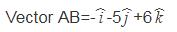Vector AB is normal to the required plane
⇒ Directions of normal (−1,−5,6)
∴ Equation ,−x−5y+6z=k
Point (2,−3,1) passes through the plane,
∴ −2+15+6=k⇒k=19
∴ −x−5y+6z = 19
x+5y−6z+19 = 0

QUESTION: 5

If the sum of the squares of the distances of a point from the three coordinate axes be 36, then its distance from the origin is

Solution:

Let (x,y,z) be the point.
Given sum of the squares of distance from point to the axes is 36.
⇒(x2+y2)+(y2+z2)+(z2+x2)=36
⇒2(x2+y2+z2)=36⇒x2+y2+z2=18
So the distance of the point from the origin is =3(2)1/2

QUESTION: 6

The locus of a point P which moves such that PA2 – PB2 = 2k2 where A and B are (3, 4, 5) and (–1, 3, –7) respectively is

Solution:
QUESTION: 7

The equation of the plane passing through the point (1, – 3, –2) and perpendicular to planes x + 2y + 2z = 5 and 3x + 3y + 2z = 8, is

Solution:

The normals to the planes x+2y+2z=5 and 3x+3y+2z=8 are their respective unit vectors ie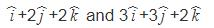Since the required plane is perpendicular to the planes
x+2y+2z=5 and 3x+3y+2z=8,
its normal would be perpendicular to the normals to the planes
The cross product of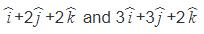is normal to the required plane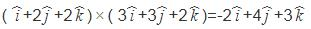The equation of the required plane would be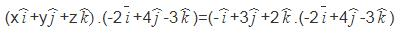-2x+4y-3z=2+12-6 ie 2x-4y+3z-8 = 0

QUESTION: 8

A variable plane passes through a fixed point (1, 2, 3). The locus of the foot of the perpendicular drawn from origin to this plane is

Solution:

α(x − α) + β(y − β) + γ(z − γ) = 0
α(1 − α) + β (2 − β) + γ ( 3 − γ) = 0
α + 2β + 3γ = α+ β+ γ2
α+ β+ γ− α − 2β − 3γ = 0
x+ y+ z− x − 2y− 3z = 0

QUESTION: 9

The reflection of the point (2, –1, 3) in the plane 3x – 2y – z = 9 is

Solution:

line AB = (x-2)/3 = (y+1)/-2 = (z-3)/-1 = λ
(x,y,z)=(3λ+2,−2λ−1,−λ+3)
3x−2y−z=9
3(3λ+2)−2(−2λ−1)−3+λ=9
9λ+6+4λ+2−3+λ=9
14λ=4
λ=2/7
C(x,y,z)=(207, -117, 19/7)
A(2,-1,3)
C is MP of A and B
C= (A+B)/2,    B= (2C-A)
B=(26/7, −22/7+1, 38/7−3)
B=(26/7,−15/7,17/7)

QUESTION: 10

The distance of the point (–1, –5, –10) from the point of intersection of the line,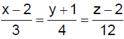and the plane, x – y + z = 5, is

Solution:
QUESTION: 11

The distance of the point (1, –2, 3) from the plane x – y + z = 5 measured parallel to the line,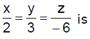Solution:
QUESTION: 12

The straight l ines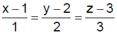and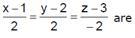Solution:
QUESTION: 13

If plane cuts off intercepts OA = a, OB = b, OC = c from the coordinate axes, then the area of the triangle ABC equal to

Solution:

=AC = −ai^ + ck^
AB = −ai^ + bj^
Area of △ABC= ½|AB × AC∣
|AB × AC∣ =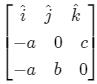−(bc)i^− (ac)j^ − (ab)k^
∣AB × AC∣ = (b2c2 + a2c2 + a2b2)1/2
Area = 1/2(a2b2 + b2c2 + c2a2)1/2

QUESTION: 14

A point moves so that the sum of the squares of its distances from the six faces of a cube given by x = ± 1, y = ± 1, z = ± 1 is 10 units. The locus of the point is

Solution:

Let P(x,y,z) be any point on the locus, then the distances from the six faces are ∣x+1∣, ∣x−1∣, ∣y−1∣, ∣z−1∣
According to the given condition, we have
∣x+1∣2 + ∣x−1∣2 + ∣y+1∣2 + ∣y−1∣2 + ∣z+1∣2+ ∣z−1∣2=10
⇒ 2(x2+y2+z2)=10−6=4
⇒ x+ y+ z= 2

QUESTION: 15

A variable plane passes through a fixed point (a, b, c) and meets the coordinate axes in A, B, C. Locus of the point common to the planes through A, B, C and parallel to coordinate plane, is

Solution:

Let equation of plane is
x/α + y/β + z/γ = 1.............(1)
Plane passes through point (a,b,c)
a/α + b/β + c/γ = 1................(2)
Again plane meets the coordinate points.
Coordinates of points are (α,0,0)
Coordinates of point B are (0, β, 0) and
coordinates of point C are (0, 0, γ)
∴ Equation of planes, parallel to coordinate axis and passing through
Point A is x = α …..(3)
Point B is y = β …..(4)
Point C is z = γ …..(5)
∴ Locus of the point of intersection is
a/x + b/y + c/z = 1

QUESTION: 16

Two systems of rectangular axes have same origin. If a plane cuts them at distances a, b, c and a1, b1, cfrom the origin, then

Solution:
QUESTION: 17

The angle between the plane 2x – y + z = 6 and a plane perpendicular to the planes x + y + 2z = 7 and x – y = 3 is

Solution:
QUESTION: 18

The non zero value of ‘a’ for which the lines 2x – y + 3z + 4 = 0 = ax + y – z + 2 and x – 3y + z = 0 = x + 2y + z + 1 are co-planar is

Solution:

2x - y +3z + 4 = 0
x - 3y + z = 0
x + 2y + z + 1 = 0
x = 12/5
y = -1/5
z= -3
this point also satisfied by
ax - y + z + 2 = 0
a(12 / 5) - (-1/5) + (-3) = 0
⇒ 12a/5 + 1/5 -3 = 0
a= -2

QUESTION: 19

If the lines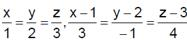and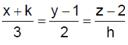are concurrent then

Solution:

Let the point of intersection is (λ, 2λ, 3λ).
Clearly, λ = 3μ + 1, 2λ = 2 – μ
Solving, we get λ = 1, μ = 0
Hence, the point of intersection is (1, 2, 3).
Therefore, (1+k)/3 = (2-1)/2 = (3-2)/h
= (1+k)/3 = 1/2 = 1/h
⇒ h = 2   k = 1/2

QUESTION: 20

The coplanar points A, B, C, D are (2 – x, 2, 2), (2, 2 – y, 2), (2, 2, 2 – z) and (1, 1, 1) respectively. Then

Solution:

We have four coplanar points.
The three vectors connecting two of them at a time are thus coplanar.
⇒{(−x, y, 0) (-x, 0, z) (1−x, 1, 1)} = 0
⇒−x (−z) −y (−x−z+xz)+0=0
⇒xz + xy + yz = xyz
⇒1/y + 1/z + 1/x = 1

QUESTION: 21

The direction ratios of a normal to the plane through (1, 0, 0), (0, 1, 0), which makes an angle of π/4 with the plane x + y = 3 are

Solution:
QUESTION: 22

Let the points A(a, b, c) and B(a', b', c') be at distances r and r' from origin. The  line AB passes through origin when

Solution:
QUESTION: 23

Let L be the line of intersection of the planes 2x + 3y + z = 1 and x + 3y + 2z = 2. If L makes an angle ? with the positive x-axis, the cos α equals

Solution:
QUESTION: 24

If a line makes an angle of π/4 with the positive directions of each of x-axis and y-axis, then the angle that the line makes with the positive direction of the z-axis is

Solution:
QUESTION: 25

If the angle θ between the line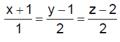and the plane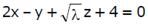is such that sinθ = 1/3 The value of λ is

Solution:
QUESTION: 26

A line makes the same angle θ with each of the x and z-axis. If the angle θ, which it makes with y-axis is such that sin2 β = 3 sin2 θ, then cos2θ equals

Solution:
QUESTION: 27

Distance between two parallel planes 2x + y + 2z = 8 and 4x + 2y + 4z + 5 = 0 is

Solution:

Let x1, y1, z1 be any point on the plane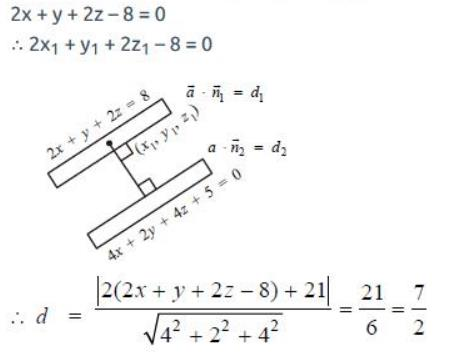QUESTION: 28

A line with direction cosines proportional to 2, 1, 2 meets each of the lines x = y + a = z and x + a = 2y = 2z. The co-ordinates of each of the points of intersection are given by

Solution:

The co-ordinates of any point on L1 in terms of parameter r1 are given by,x=y+a=z=r1
⇒x=r1,y=r1 - a,z=r1....(1)
Similarly the co-ordinates of any point on L2 in terms of parameter 2r2 are given by
⇒x+a=2y=2z=2r2
⇒ x=2r2−a,y=r2, z=r2...(2)
Let 'A' be a point on L1 and 'B' be a point on L2
​Using (1) and (2), the direction ratios of AB are
2r2−a−r1,r2−r1+a,r2−r1
​If the above line is same as the line whose direction cosines are proportional to (2,1,2) as given in the question, then
(2r2−r1−a)/2= (r2−r1+a)/1 = (r2−r1)/2
Solving the first two of the above equation, we get r1=3a
Again solving the last two, we get r2=a
Using these values in (1) and (2), we get the coordinates of points as

(3a,2a,3a) and (a,a,a).

QUESTION: 29

The lines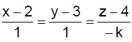and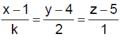are coplanar if

Solution:

(x-x1)a1 = (y-y1)b1 = (z-z1)c1 &
(x-x2)a2 = (y-y2)b2 = (z-z2)c2 are coplanar if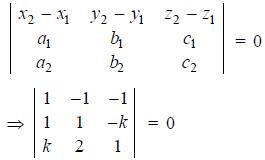k2 + 3k = 0
k = 0 or -3

QUESTION: 30

The equation of plane which meet the co-ordinate axes whose centroid is (a, b, c)

Solution:

A(a,0,0),B(0,b,0),C(0,0,c) are the points on coordinate axis and centriod (α,β,γ).
According to centroid formula,
α= a/3
​a=3α
b=3β
c=3γ
Equation of plane is,x/a+y/b+z/c =1
x/α+y/β+z/γ=3